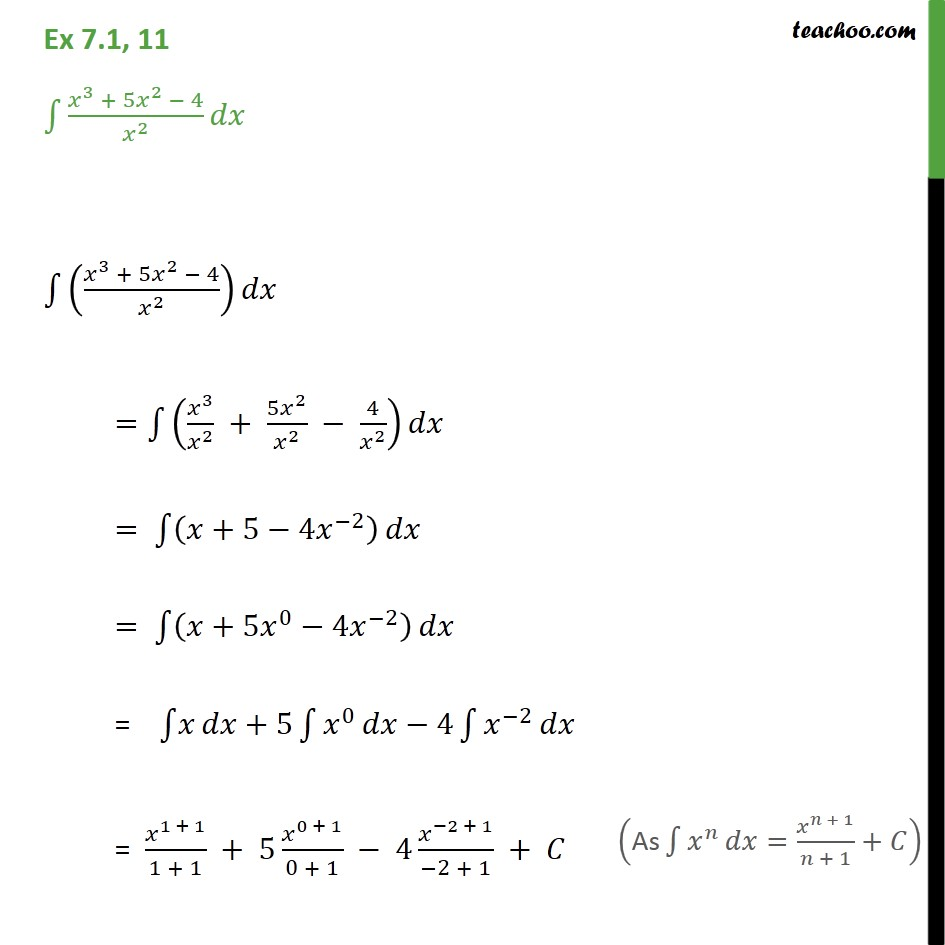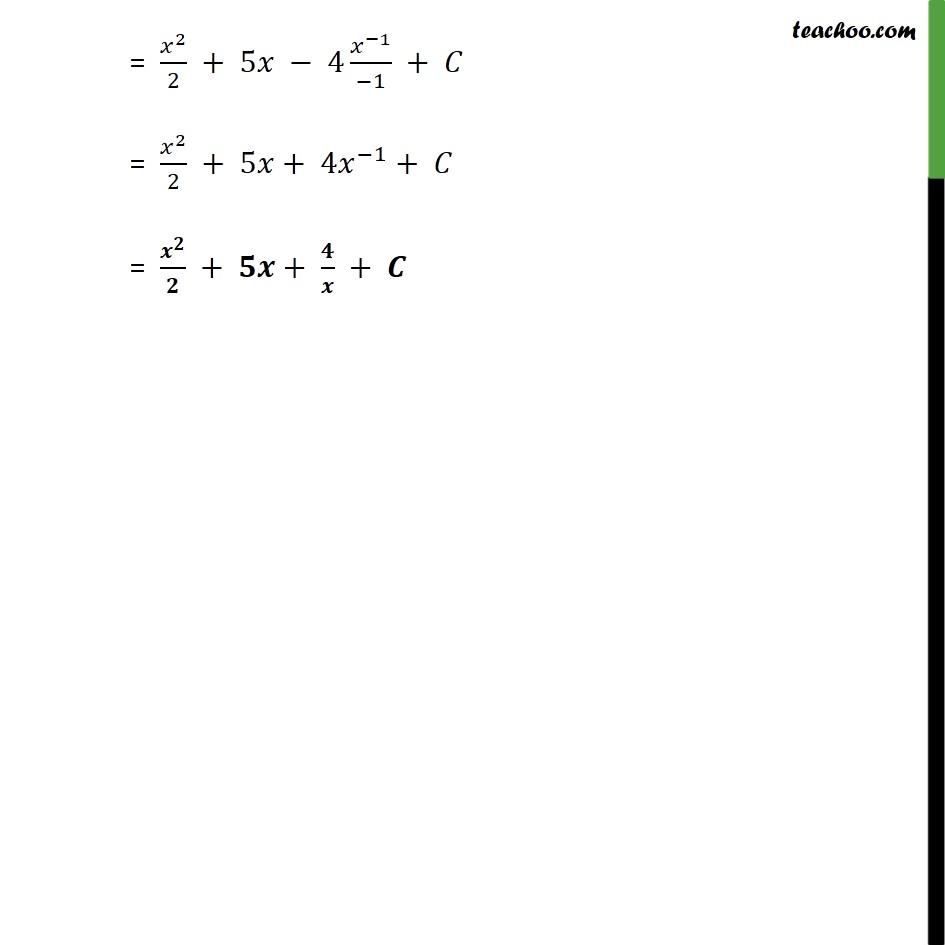Ex 7.1

Chapter 7 Class 12 Integrals
Serial order wiseLearn in your speed, with individual attention - Teachoo Maths 1-on-1 Class

### Transcript

Ex 7.1, 11 3 + 5 2 4 2 3 + 5 2 4 2 = 3 2 + 5 2 2 4 2 = +5 4 2 = +5 0 4 2 = +5 0 4 2 = 1 + 1 1 + 1 + 5 0 + 1 0 + 1 4 2 + 1 2 + 1 + = 2 2 + 5 4 1 1 + = 2 2 + 5 + 4 1 + = + + +